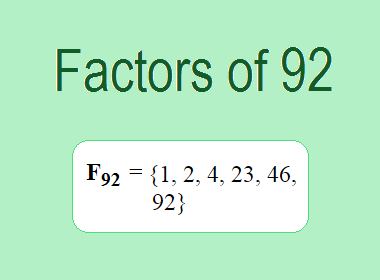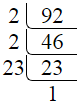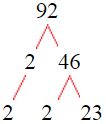# Factors of 92The factors of 92 are 1, 2, 4, 23, 46, and 92 i.e. F92 = {1, 2, 4, 23, 46, 92}. The factors of 92 are all the numbers that can divide 92 without leaving a remainder.

We can check if these numbers are factors of 92 by dividing 92 by each of them. If the result is a whole number, then the number is a factor of 92. Let's do this for each of the numbers listed above:

·        1 is a factor of 92 because 92 divided by 1 is 92.

·        2 is a factor of 92 because 92 divided by 2 is 46.

·        4 is a factor of 92 because 92 divided by 4 is 23.

·        23 is a factor of 92 because 92 divided by 23 is 4.

·        46 is a factor of 92 because 92 divided by 46 is 2.

·        92 is a factor of 92 because 92 divided by 92 is 1.

## How to Find Factors of 92?

1 and the number itself are the factors of every number. So, 1 and 92 are two factors of 92. To find the other factors of 92, we can start by dividing 92 by the numbers between 1 and 92. If we divide 92 by 2, we get a remainder of 0. Therefore, 2 is a factor of 92. If we divide 92 by 3, we get a remainder of 2. Therefore, 3 is not a factor of 92.

Next, we can check if 4 is a factor of 92. If we divide 92 by 4, we get a remainder of 0. Therefore, 4 is also a factor of 92. We can continue this process for all the possible factors of 92.

Through this process, we can find that the factors of 92 are 1, 2, 4, 23, 46, and 92. These are the only numbers that can divide 92 without leaving a remainder.

********************

********************

## Properties of the Factors of 92

The factors of 92 have some interesting properties. One of the properties is that the sum of the factors of 92 is equal to 168. We can see this by adding all the factors of 92 together:

1 + 2 + 4 + 23 + 46 + 92 = 168

Another property of the factors of 92 is that the prime factors of 92 are 2 and 23 only.

## Applications of the Factors of 92

The factors of 92 have several applications in mathematics. One of the applications is in finding the highest common factor (HCF) of two or more numbers. The HCF is the largest factor that two or more numbers have in common. For example, to find the HCF of 92 and 69, we need to find the factors of both numbers and identify the largest factor they have in common. The factors of 92 are 1, 2, 4, 23, 46, and 92. The factors of 69 are 1, 3, 23, and 69. The largest factor that they have in common is 23. Therefore, the HCF of 92 and 69 is 23.

Another application of the factors of 92 is in prime factorization. Prime factorization is the process of expressing a number as the product of its prime factors. The prime factors of 92 are 2 and 23, since these are the only prime numbers that can divide 92 without leaving a remainder. Therefore, we can express 92 as:

92 = 2 × 2 × 23

We can do prime factorization by division and factor tree method also. Here is the prime factorization of 92 by division method,92 = 2 × 2 × 23

Here is the prime factorization of 92 by the factor tree method,92 = 2 × 2 × 23

## Conclusion

The factors of 92 are the numbers that can divide 92 without leaving a remainder. The factors of 92 are 1, 2, 4, 23, 46, and 92. The factors of 92 have some interesting properties, such as having a sum of 168. The factors of 92 have several applications in mathematics, such as finding the highest common factor and prime factorization.